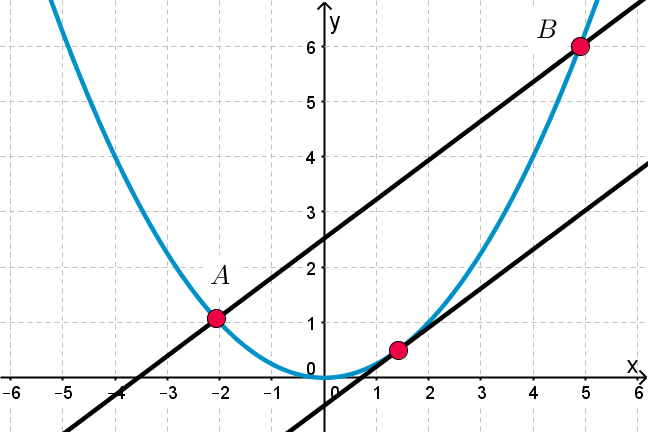4.7

## UNSW SydneyA secant and a tangent to a parabola

# Secants and tangents of a parabola

A secant of a parabola is a line, or line segment, that joins two distinct points on the parabola. A tangent is a line that touches the parabola at exactly one point. Finding tangents to curves is historically an important problem going back to Fermat, and is a key motivator for the differential calculus.

In this step we will see how to algebraically find both secants and tangents to a parabola. So this section gives an alternative algebraic perspective on calculus concepts!

## A parametrization of the parabola

The standard parabola $\normalsize{y=ax^2}$ has a nice parametrization of the form

For any value of $\normalsize{t}$, this formula for $\normalsize{A}$ gives us a point lying on the parabola.

In the figure we see the parabola $\normalsize{y=\frac{1}{4}x^2}$. Note that as $\normalsize{t}$ varies, the $\normalsize{x}$- coordinate of $\normalsize{A}$ moves linearly, while the $\normalsize{y}$- coordinate moves quadratically.## Secant of a parabola

Now suppose that we have two points $\normalsize{A=[t,at^2]}$ and $\normalsize{B=[u,au^2]}$ on the parabola $\normalsize{y=ax^2}$. The line $\normalsize{AB}$ joining $\normalsize{A}$ and $\normalsize{B}$ has slope

and so it has an equation of the form $\normalsize{y=a(u+t)x+b}$. To determine the $\normalsize{y}$-intercept $\normalsize{b}$, we may substitute in the coordinates $\normalsize{[t,at^2]}$. We get that $\normalsize{b=-aut}$ so the equation of the secant is

Q1 (E): What is the equation of the secant through the points on the parabola $\normalsize{y=x^2}$ whose $\normalsize{x}$- coordinates are $\normalsize{3}$ and $\normalsize{5}$ ?

## Tangent to a parabola

What happens to our formula for the secant when $\normalsize{t}$ and $\normalsize{u}$ are very close? Better yet, what happens when we set $\normalsize{t=u}$, so that $\normalsize{A=B}$? This is actually a very interesting situation that rewards careful examination. We are parachuting into the hallowed halls of calculus using algebra!

In this case the equation of the secant becomes the equation of the tangent to the parabola: starting with the equation of the secant ${\normalsize y=a(t+u)x-atu}$ and substituting $\normalsize u=t$ gives:

Note that in particular the slope of the tangent line to the point with $\normalsize{x}$-coordinate $\normalsize{t}$ is $\normalsize{2at}$. This number is usually called the derivative of the function at the point $\normalsize{x}$, and in calculus courses is introduced following a study of limits. Our purely algebraic techniques allowed us to find the equation of the tangent to the parabola $\normalsize{y=ax^2}$. Since any other parabola is just a translate of such, we see that we can deal with secants and tangents for general parabolas, without limits!

Q2 (E): What is the equation of the tangent to the parabola $\normalsize{y=\frac{1}{4} x^2}$ at the point $\normalsize{[3,9/4]}$?## Two properties of the parabola

Here are two interesting challenges for you. Let’s see if we can derive some interesting aspects of the geometry of the parabola. The second one will require some thorough thinking. You will need to know that two lines are perpendicular precisely when their slopes multiply to $\normalsize{-1}$.

Q3 (M): Consider the points $\normalsize{A=[t,at^2]}$ and $\normalsize{B=[u,au^2]}$ on the parabola $\normalsize{y=ax^2}$. Show that the two tangents to the parabola at $\normalsize{A}$ and $\normalsize{B}$ meet at $\normalsize{P=[(u+t)/2,aut]}$.Notice that the $\normalsize x$- coordinate of $\normalsize P$ is halfway between $\normalsize A$ and $\normalsize B$, and does not depend on the particular parabola.

Q4 (C): Show that the two tangents to the parabola $\normalsize{y=x^2}$ through the points $\normalsize{A=[t,t^2]}$ and $\normalsize{B=[u,u^2]}$ are perpendicular precisely when the line $\normalsize{AB}$ passes through the focus of the parabola.A1. The secant is the line ${\normalsize y = 8x - 15}$.

A2. Apply the formula ${\normalsize y=2atx-at^2}$ with ${\normalsize a=1/4}$ and ${\normalsize t=3}$ to get the tangent: ${\normalsize y=\frac32 x-\frac94}$.

A3. Solve the equations $\normalsize{y=2atx-at^2}$ and $\normalsize{y=2aux-au^2}$ simultaneously.

A4. You can do it!